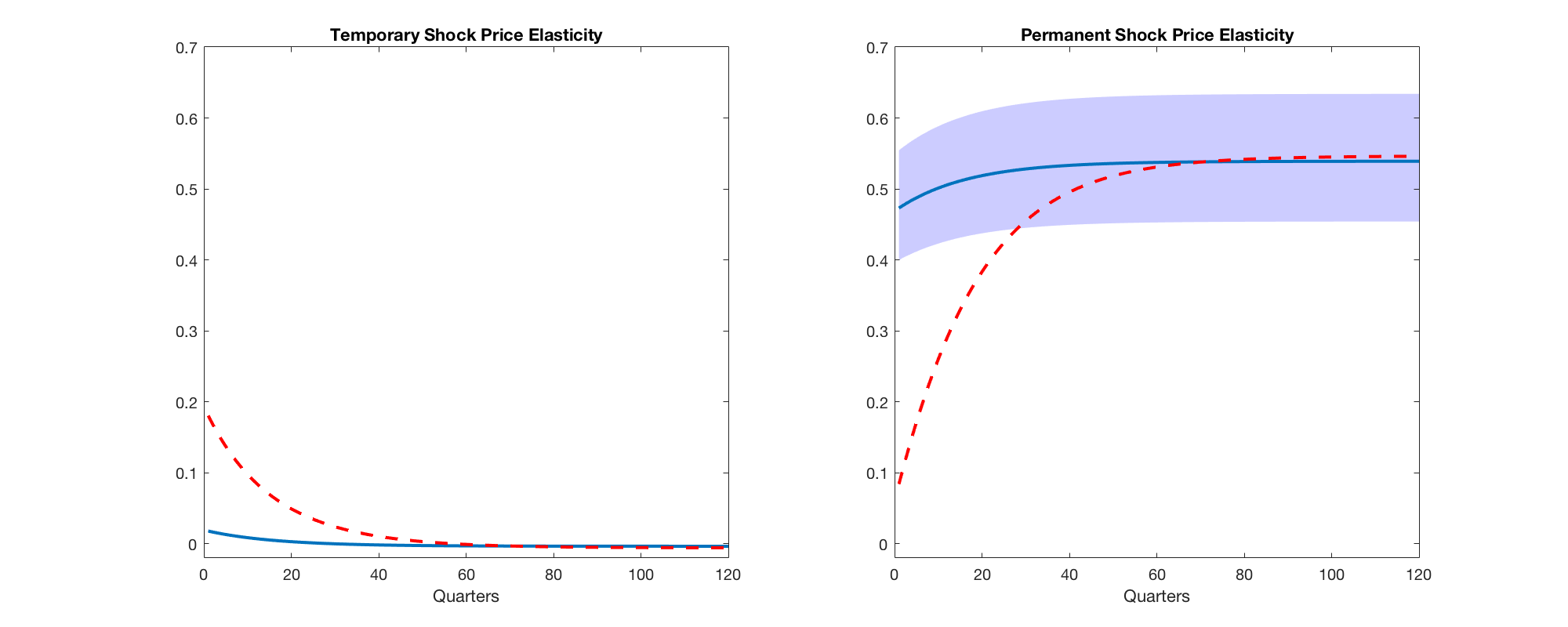Computing Shock Elasticities for Bansal and Yaron (2004)

In this MATLAB live script, we will recreate the shock elasticities plots in this webpage. The script uses the toolbox developed by MFM (download here). If you wish to run the MATLAB script directly without following the detailed steps, simply run the BansalYaron.m file in the toolbox.
The model, adapted from Bansal and Yaron (2004), has two state variables that follow stochastic processes:Where aggregate consumption follows: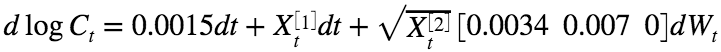Please note that the parameter settings and specific continuous-time specification comes from the handbook chapter of Hansen, Heaton, Lee, Roussanov (2007) Intertemporal Substitution and Risk Aversion to well approximate the dynamics used by Bansal and Yaron (2004). As in Hansen (2012), we transform two of the shocks to the macroeconomy in order that one is permanent and the other is transitory. Furthermore, the intertemporal elasticity parameter is unity and the risk aversion parameter is 8.

Step 1: Input parameters

Suppose that the state dynamics are specified byandand the consumption dynamics take the following form: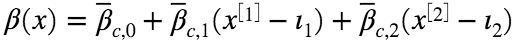and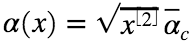We can recast the model by setting,,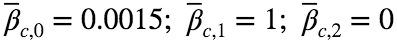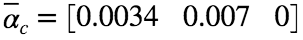In MATLAB, this corresponds to:
muX = [-0.021 0; 0 -0.013];
iota = [0 -1]';
sigma = [0.00031 -0.00015 0; 0 0 -0.038];
beta0 = 0.0015; beta1 = 1; beta2 = 0;
alpha = [0.0034 0.007 0];
delta = 0; gamma = 8;
Note that we have chosen parameters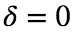and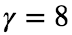.

Step 2: Configure inputs for the toolbox

First, we plan to initialize 100 grid points for each of the two state variables. For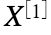, we initialize from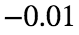to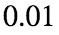. For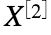, we initialize from 0 to 2.
n1 = 100; n2 = 100;
[X,Y] = ndgrid(linspace(-0.01,0.01,n1), linspace(0,2,n2));
Now, we will configure the drifts and volatilities of the state variables.
%%%Create function handle for the drifts
model.muX = @(x) (x + iota') * muX;
%%%Create function handles for the vols
sigmaX1Func = @(x) sqrt(x(:,2)) * sigma(1,:);
sigmaX2Func = @(x) sqrt(x(:,2)) * sigma(2,:);
model.sigmaX = {sigmaX1Func, sigmaX2Func};
We then configure the drift and volatility of consumption.
%%%Create function handles for drift and vol of consumption process
model.muC = @(x) x(:,1) + beta0;
model.sigmaC = @(x) sqrt(x(:,2)) * alpha;
Next, we will configure the drift and volatility of the SDF for the recursive utility function. Note that the SDF is given by:WhereWhen we take logs:where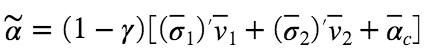. Note that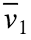and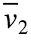are computed bywhere A, B, and C in the quadratic formula are given by%%%Create function handles for drift and vol of EZ utility function
v1 = - beta1 / (muX(1,1) - delta);
A = (1 - gamma) / 2 * ( sum(sigma(1,:) .* sigma(1,:)) );
B = -delta + muX(2,2) + (1 - gamma) * alpha * sigma(2,:)' ...
+ 2 * v1 * (1 - gamma) / 2 * sigma(1,:) * sigma(2,:)';
C = muX(1,2) * v1 + beta2 + (1 - gamma) * alpha * sigma(1,:)' * v1 ...
+ (1 - gamma) / 2 * ( sigma(1,:) * sigma(1,:)' * v1^2 - alpha * alpha');
v2 = (-B - sqrt(B^2 - 4*A*C)) / (2 * A);
alphaTilde = (1 - gamma) * (sigma(1,:) * v1 + sigma(2,:) * v2 + alpha);
model.muS = @(x) (-delta - 1 * (beta0 + beta1 * x(:,1) + beta2 * ( x(:,2) - 1) ) ...
- alphaTilde * alphaTilde' / 2 * x(:,2));
model.sigmaS = @(x) sqrt(x(:,2)) * (alphaTilde - 1 * alpha);
At this step, we will configure the boundary conditions and the time settings. For simplicity, we will use zero first derivatives as the boundary conditions for the Feynman-Kac formula. We want to compute shock elasticities for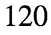quarters. As the units are monthly, we will use time step 1 and compute for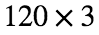periods, which are equivalent to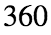months orquarters.
Furthermore, since this problem is not computationally intensive, we will not use Pardiso, and we will tell the toolbox to compute price elasticities given the SDF.
%%%%Configure the bc and time settings
bc = struct; bc.a0 = 0; bc.first = [1 1]; bc.second = [0 0];
bc.third = [0 0]; bc.level = [0 0];
bc.natural = false;
model.T = 120 * 3 ; model.dt = 1;
optArgs.usePardiso = false;
optArgs.priceElas = true;

Step 3: Compute stationary density

At this point, we are almost ready to compute shock elasticities. However, we need to pick the starting points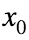. We will pick starting points by fixingat the mean and select three points from the 10th, 50th, and 90th percentiles of. Although we know the theoretical values, for the sake of illustration, we will simulate to get the stationary density.
g_bar = 0.0; s_bar = 1.0;
gStd = s_bar * sqrt( sum(sigma(1,:).^2) / (2 * -muX(1,1)));
shape = 2 * -muX(2,2) * s_bar / ( sum(sigma(2,:).^2) );
rate = 2 * -muX(2,2) / ( sum(sigma(2,:).^2) );
sStd = sqrt(shape/(rate^2));
drifts = {@(x) (x(1,:) + iota') * muX(:,1), @(x) (x(:,2) + iota') * muX(:,2) };
dt = 1/255;
T = round(5000 / 10);
hist0 = zeros(10, 2 );
hist0(:,1) = normrnd(g_bar, gStd,10,1); hist0(:,2) = gamrnd(shape,1/rate,10,1);
%%Simulate
tic(); hists = simStatDent( {linspace(-0.01,0.01,100), linspace(0,2,100)}, hist0, dt, T, drifts, model.sigmaX); toc()
Elapsed time is 352.460287 seconds.
Note that the actual time taken in your MATLAB terminal is much shorter, as the live script is slower than the actual MATLAB console. We burned the first 20% of the simulated data and find the desired percentiles.
burnRate = 0.2;
burned = cellfun(@(x) x(round( burnRate * size(x,1)):end,:), hists, 'UniformOutput', false);
alldata = cat(1, burned{:});
x2Percentiles = prctile(alldata(:,2),[10, 50, 90]);
x0 = [[0 0 0]' x2Percentiles'];
We have computed the stationary density. We now plot the unconditional distributions of the two state variables and highlight the desired percentiles of both state variables. In addition, we overlay the theoretical distribution (a gamma distribution) ofon top of the simulated distribution.
%%Plot distribution
totalPoints = size(alldata,1);
figure('pos', [10 10 1000 400]);
[vals, edges] = histcounts( alldata(:, 1) );
centers = edges(1:end-1)+ diff(edges)/2;
subplot(1,2,1)
bar(centers, vals ./ size( alldata(:, 1) , 1));
hold on
h1 = plot([x1Percentiles(1) x1Percentiles(1)],ylim,'r', 'LineWidth', 1.5);
h2 = plot([x1Percentiles(2) x1Percentiles(2)],ylim, '--r', 'LineWidth', 1.5);
h3 = plot([x1Percentiles(3) x1Percentiles(3)],ylim, ':r', 'LineWidth', 1.5);
legend([h1 h2 h3],{'10th Pct', '50th Pct', '90th Pct'})
xlabel('$$X^{}$$', 'interpreter', 'latex'); title('Unconditional Distribution of $$X^{}$$','interpreter','latex') ;
[vals, edges] = histcounts( alldata(:, 2) );
centers = edges(1:end-1)+ diff(edges)/2;
subplot(1,2,2)
bar(centers, vals ./ size( alldata(:, 2) , 1));
hold on
h1 = plot([x2Percentiles(1) x2Percentiles(1)],ylim,'r', 'LineWidth', 1.5);
h2 = plot([x2Percentiles(2) x2Percentiles(2)],ylim, '--r', 'LineWidth', 1.5);
h3 = plot([x2Percentiles(3) x2Percentiles(3)],ylim, ':r', 'LineWidth', 1.5);
%%Get analytical solution for the second state variable
scale = 1 /rate;
x = gaminv((0.001:0.001:0.999),shape,scale);
y = gampdf(x,shape,scale);
plot(x,y/100, 'LineWidth', 1.5)
legend([h1 h2 h3],{'10th Pct', '50th Pct', '90th Pct'})
hold off
xlabel('$$X^{}$$', 'interpreter', 'latex'); title('Unconditional Distribution of $$X^{}$$','interpreter','latex') ;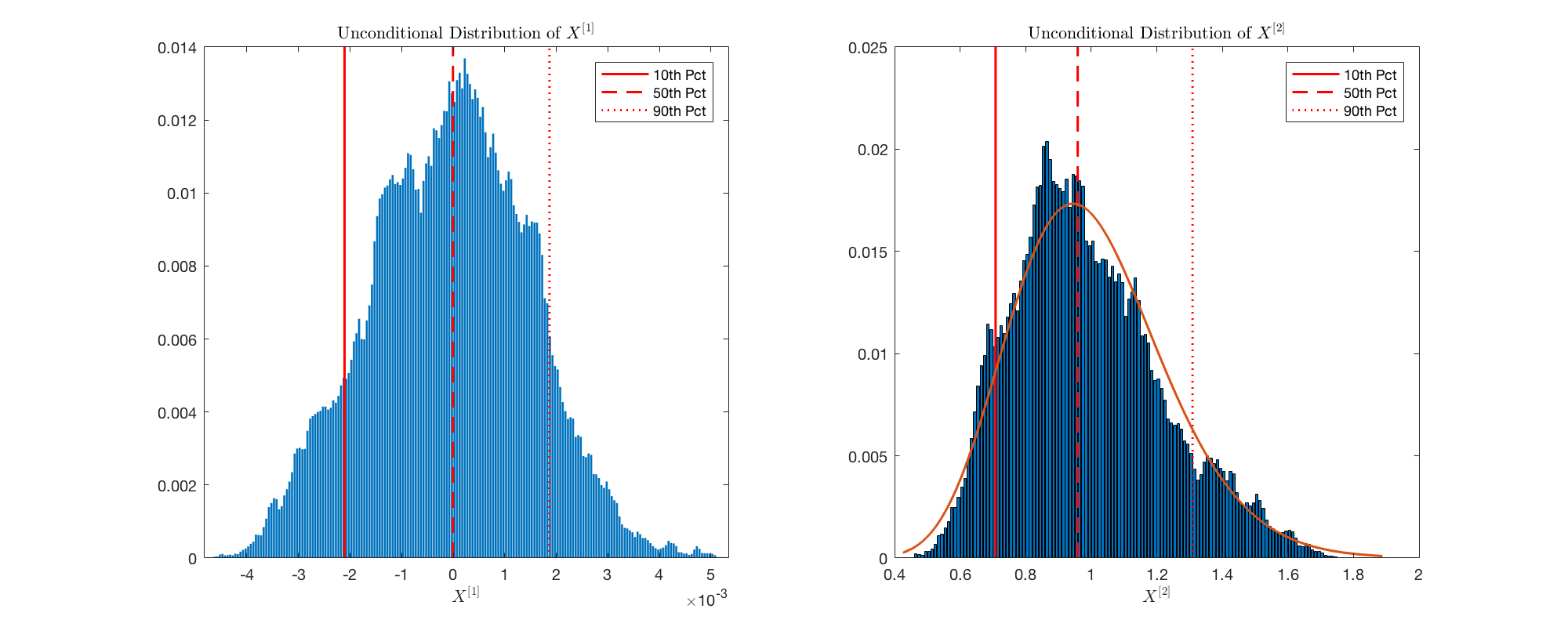Step 4: Compute Shock Elasticities for Recursive and Power Utility

We are now ready to compute shock elasticities. First, we compute the shock elasticities of the recursive utility function.
tic(); [expoElas, priceElas] = computeElas( [X(:) Y(:)], model, bc, x0, optArgs); toc()
COMPUTING SHOCK EXPOSURE ELASTICITIES ...Constructing matrix... ...Finished constructing matrix... ...Factorizing matrix... ...Finished factorizing matrix... ...Solving for expectation(phi)... ...Finished solving for expectation(phi)... Finished solving Feynman Kac equation... Finished interpolating... Finished computing first type. Computing second type... Finished computing second type. COMPUTING SHOCK PRICE ELASTICITIES ...Constructing matrix... ...Finished constructing matrix... ...Factorizing matrix... ...Finished factorizing matrix... ...Solving for expectation(phi)... ...Finished solving for expectation(phi)... Finished solving Feynman Kac equation... Finished interpolating... Finished computing first type. Computing second type... Finished computing second type. Elapsed time is 24.557880 seconds.
Now we configure the SDF for power utility. Note that the SDF for power utility is given by:Taking logs yields%%%%Compute shock elasticities, this time for power utility
model.muS = @(x) - delta - gamma * model.muC(x);
model.sigmaS = @(x) sqrt( x(:,2) ) * ( - gamma * alpha);
tic(); [expoElasPower, priceElasPower] = computeElas( [X(:) Y(:)], model, bc, x0, optArgs); toc()
COMPUTING SHOCK EXPOSURE ELASTICITIES ...Constructing matrix... ...Finished constructing matrix... ...Factorizing matrix... ...Finished factorizing matrix... ...Solving for expectation(phi)... ...Finished solving for expectation(phi)... Finished solving Feynman Kac equation... Finished interpolating... Finished computing first type. Computing second type... Finished computing second type. COMPUTING SHOCK PRICE ELASTICITIES ...Constructing matrix... ...Finished constructing matrix... ...Factorizing matrix... ...Finished factorizing matrix... ...Solving for expectation(phi)... ...Finished solving for expectation(phi)... Finished solving Feynman Kac equation... Finished interpolating... Finished computing first type. Computing second type... Finished computing second type. Elapsed time is 27.743385 seconds.

Step 5: Plot Results

All the computations are finished. We now plot the results.
%%Plot exposure elasticities
figure('pos', [10 10 1000 400])
subplot(1,2,1);
y1 = ( exp(3*expoElas{2}.firstType(:,2))-1 );
x = linspace(1,model.T/3, model.T);
fillX=[x,fliplr(x)];
fillY=[exp(3*expoElas{3}.firstType(:,2))'-1, fliplr(exp(3*expoElas{1}.firstType(:,2))'-1)];
fill(fillX,fillY,'b', 'facealpha', .2, 'EdgeColor','None');
hold on
plot(x,y1, 'LineWidth', 2);
hold off
xlim([0 120])
ylim([-.001 0.07])
xlabel('Quarters')
title('Temporary Shock Exposure Elasticity')
subplot(1,2,2);
y1 = ( exp(3*expoElas{2}.firstType(:,1))-1 );
x = linspace(1,model.T/3, model.T);
fillX=[x,fliplr(x)];
fillY=[exp(3*expoElas{3}.firstType(:,1))'-1, fliplr(exp(3*expoElas{1}.firstType(:,1))'-1)];
fill(fillX,fillY,'b', 'facealpha', .2, 'EdgeColor','None');
hold on
plot(x,y1, 'LineWidth', 2);
hold off
xlim([0 120])
ylim([-.001 0.07])
xlabel('Quarters')
title('Permanent Shock Exposure Elasticity')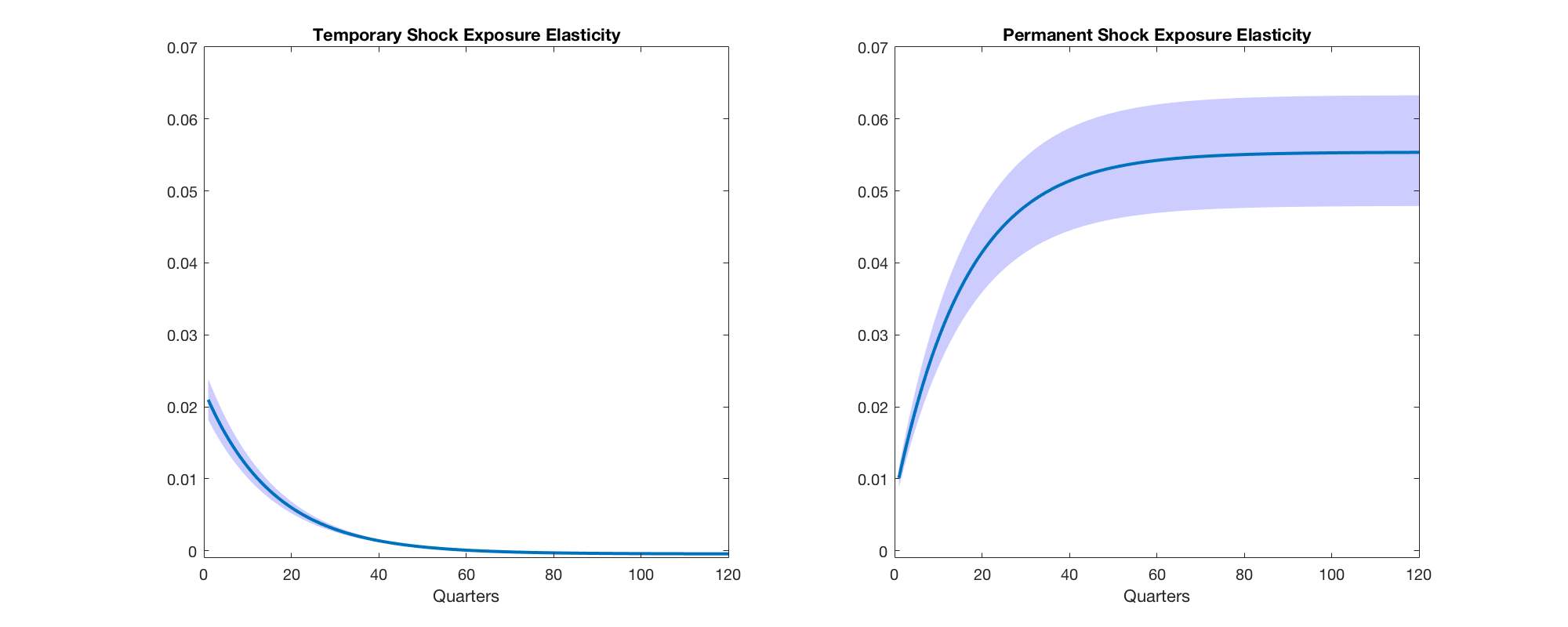%%Plot price elasticities
figure('pos', [10 10 1000 400])
subplot(1,2,1);
y1 = ( exp(3*priceElas{2}.firstType(:,2))-1 );
x = linspace(1,model.T/3, model.T);
fillX=[x,fliplr(x)];
fillY=[exp(3*priceElas{3}.firstType(:,2))'-1, fliplr(exp(3*priceElas{1}.firstType(:,2))'-1)];
fill(fillX,fillY,'b', 'facealpha', .2, 'EdgeColor','None');
hold on
plot(x,y1, 'LineWidth', 2);
plot(x, exp(3*priceElasPower{2}.firstType(:,2))-1 , 'r--', 'LineWidth', 2);
hold off
xlabel('Quarters')
title('Temporary Shock Price Elasticity')
xlim([0 120])
ylim([-.02 0.7])
subplot(1,2,2);
y1 = ( exp(3*priceElas{2}.firstType(:,1))-1 );
x = linspace(1,model.T/3, model.T);
fillX=[x,fliplr(x)];
fillY=[exp(3*priceElas{3}.firstType(:,1))'-1, fliplr(exp(3*priceElas{1}.firstType(:,1))'-1)];
fill(fillX,fillY,'b', 'facealpha', .2, 'EdgeColor','None');
hold on
plot(x,y1, 'LineWidth', 2);
plot(x, exp(3*priceElasPower{2}.firstType(:,1))-1 , 'r--', 'LineWidth', 2);
hold off
xlabel('Quarters')
title('Permanent Shock Price Elasticity')
xlim([0 120])
ylim([-.02 0.7])